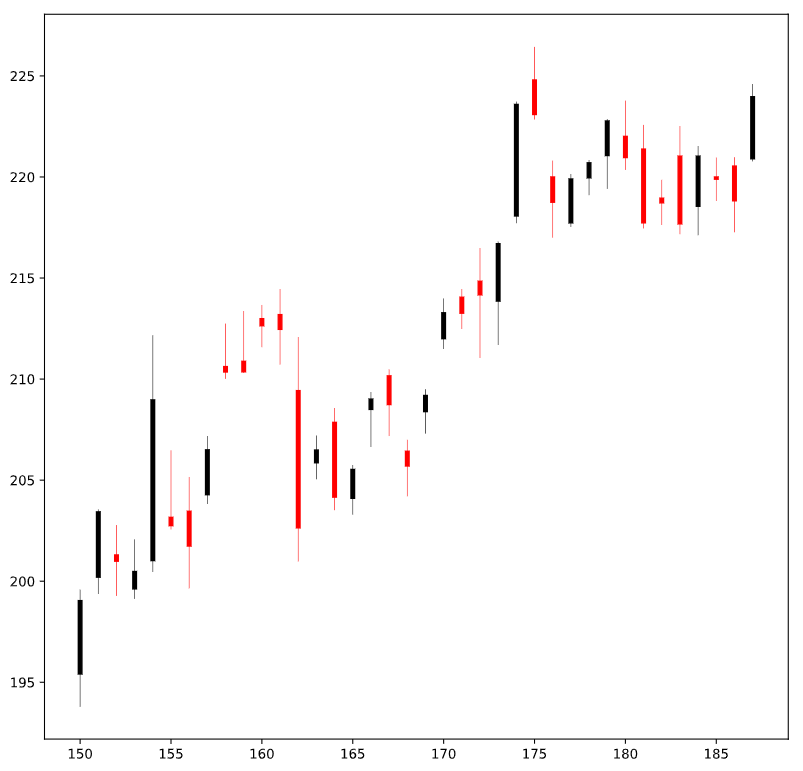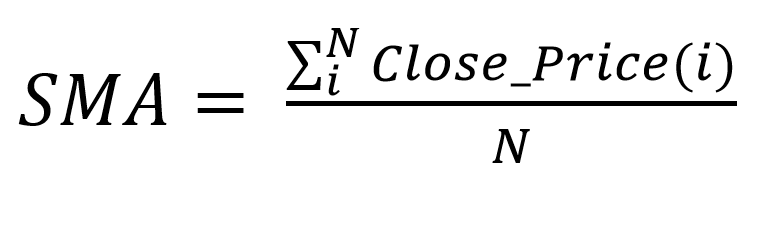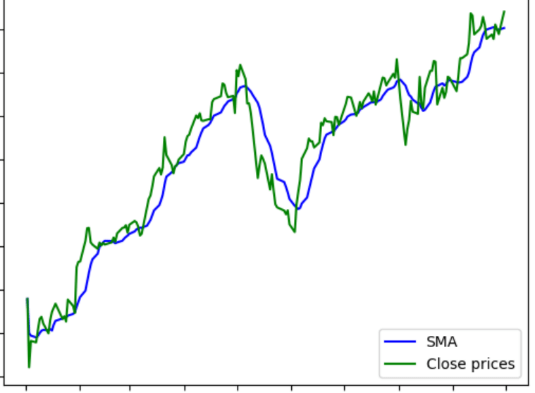# 股票价格分析：如何使用Python介绍财务和技术指标？

2021年11月11日03:05:22 发表评论 797 次浏览

Python介绍财务和技术指标 - 学习如何在 Python 中处理股票价格，了解蜡烛价格格式 (OHLC)，使用烛台图表绘制它们，以及学习使用 Python 中的 stockstats 库使用许多技术指标。

Python如何分析股票价格？金融市场以其供求规律和其他经济因素的动态势头而闻名，也是产生大量数据的地方。分析股票价格，无论你是交易差价合约（差价合约）、期货还是商品，无论你使用的是何种经纪商，都强烈建议你在进行交易时最大化你的收益，甚至是最基本的。

``pip install pandas-datareader yfinance mpl-finance stockstats``

## 从 Yahoo Finance API 导入数据

Python股票价格分析：要导入股票价格数据，我们需要指定市场指数或股票的代码，在本例中，我们将从`S&P 500`和导入数据`AAPL`

``````# import AAPL stock price
df_aapl = pdr.get_data_yahoo("AAPL", start="2019-01-01", end="2019-09-30")``````

``````# import SPY stock price
df_spy = pdr.get_data_yahoo("SPY", start="2019-01-01", end="2019-09-30")``````

``df_spy.head()``

• 开盘价是时间分辨率开始时的股票价格（1m、30m、每小时、每天等）
• 高：是从蜡烛的开始到结束达到的最高价格。
• 低：是从蜡烛的开始到结束达到的最低价格。
• 收盘是时间决议结束时股票的价格。

## 绘制股票价格

Python介绍财务和技术指标：在本节中，我们将使用matplotlibmpl_finance库来绘制`AAPL`. 首先，我们将分别绘制开盘-高-低-收盘价：

``````df_aapl[["Open", "High", "Low", "Close"]].plot()
plt.show()``````

Python如何分析股票价格？我们可以在放大的区域中清楚地看到，高价（橙色图）是上限，而低价（绿色图）是下限，这说明了本教程上一节中提到的 OHLC 格式的定义。

``````fig = plt.figure(figsize=(10, 10))
ax = plt.subplot()

plot_data = []
for i in range(150, len(df_aapl)):
row = [
i,
df_aapl.Open.iloc[i],
df_aapl.High.iloc[i],
df_aapl.Low.iloc[i],
df_aapl.Close.iloc[i],
]
plot_data.append(row)
candlestick_ohlc(ax, plot_data)
plt.show()``````以下是烛台的结构：

## 金融技术指标

Python介绍财务和技术指标：在金融方面，由于我们正在处理数字数据，因此除了检测趋势之外，依靠各种指标还可以更好地了解股票价格的走势，这对于我们打算对特定股票进行长期交易/投资非常重要.

stockstats库，我们将导入`StockDataFrame`，这是接收作为一个属性的熊猫一类`DataFrame`排序按时间和包括顺序列开-关-高-低：

``````from stockstats import StockDataFrame

stocks = StockDataFrame.retype(df_aapl[["Open", "Close", "High", "Low", "Volume"]])``````

### 简单移动平均线 (SMA)

SMA的公式如下图所示：``````plt.plot(stocks["close_10_sma"], color="b", label="SMA")
plt.plot(df_aapl.Close, color="g", label="Close prices")
plt.legend(loc="lower right")
plt.show()``````为了计算 SMA，我们按照以下格式指定属性：

`Column_Period_Indicator`

### 指数移动平均线 (EMA)

Python股票价格分析：不同的是SMA，这给相等的权重，以所有的价格无论是新的或旧的，在美国，指数移动平均线通过赋予最近(最近)的价格更大的权重来强调它们，这使得均线成为一个比均线更快检测趋势的指标。

EMA的公式如下：

``````plt.plot(stocks["close_10_sma"], color="b", label="SMA") # plotting SMA
plt.plot(stocks["close_10_ema"], color="k", label="EMA")
plt.plot(df_aapl.Close, color="g", label="Close prices") # plotting close prices
plt.legend(loc="lower right")
plt.show()``````

### 移动平均收敛/发散 (MACD)

Python如何分析股票价格？移动平均收敛/发散是趋势跟踪动量指标。该指标可以显示价格变动速度的变化，交易者可以使用它来确定趋势的方向。

MACD 的计算方法是从 12 周期 EMA 中减去 26 周期指数移动平均线 (EMA)。MACD 的 9 天 EMA 称为“信号线”，然后与 MACD 一起绘制。

``````plt.plot(stocks["macd"], color="b", label="MACD")
plt.plot(stocks["macds"], color="g", label="Signal Line")
plt.legend(loc="lower right")
plt.show()``````

## Python介绍财务和技术指标总结